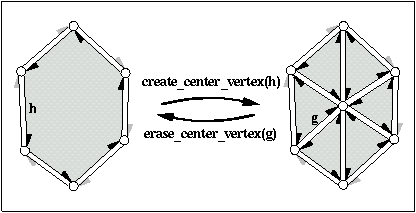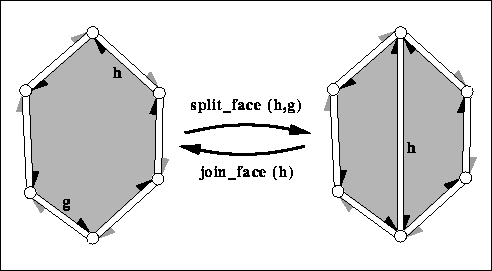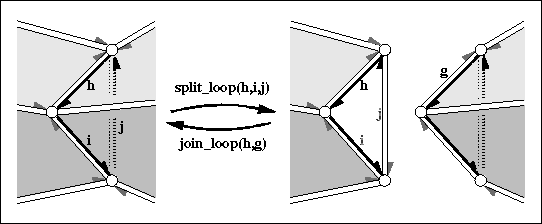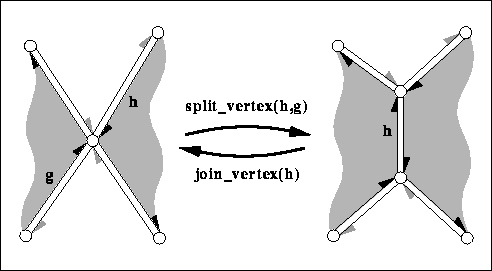CGAL 4.7 - Halfedge Data Structures
CGAL::HalfedgeDS_decorator< HDS > Class Template Reference

#include <CGAL/HalfedgeDS_decorator.h>

## Definition

The class CGAL::HalfedgeDS_items_decorator<HDS> provides additional functions for vertices, halfedges, and faces of a halfedge data structure without knowing the containing halfedge data structure.

The class CGAL::HalfedgeDS_decorator<HDS> stores a reference to the halfedge data structure and provides functions that modify the halfedge data structure, for example Euler-operators. The class CGAL::HalfedgeDS_const_decorator<HDS> stores a const reference to the halfedge data structure. It contains non-modifying functions, for example the test for validness of the data structure.

All these additional functions take care of the different capabilities a halfedge data structure may have or may not have. The functions evaluate the type tags of the halfedge data structure to decide on the actions. If a particular feature is not supported nothing is done. Note that for example the creation of new halfedges is mandatory for all halfedge data structures and will not appear here again.

CGAL::HalfedgeDS_items_decorator<HDS>
CGAL::HalfedgeDS_const_decorator<HDS>

Example

The following program fragment illustrates the implementation of the Euler operator split_vertex() for a simplified polyhedron class.

template <class Traits>
namespace CGAL {
class Polyhedron {
typedef HalfedgeDS_default<Traits> HDS;
HDS hds;
public:
// ...
HalfedgeDS_decorator<HDS> D(hds);
// Stricter preconditions than for HalfedgeDS only.
CGAL_precondition( D.get_vertex(h) == D.get_vertex(g));
CGAL_precondition( h != g);
return D.split_vertex( h, g);
}
};
}
Examples:
HalfedgeDS/hds_prog_compact.cpp, HalfedgeDS/hds_prog_compact2.cpp, HalfedgeDS/hds_prog_default.cpp, HalfedgeDS/hds_prog_edge_iterator.cpp, HalfedgeDS/hds_prog_graph.cpp, HalfedgeDS/hds_prog_graph2.cpp, HalfedgeDS/hds_prog_halfedge_iterator.cpp, and HalfedgeDS/hds_prog_vector.cpp.

## Creation

HalfedgeDS_decorator (HDS &hds)
keeps internally a reference to hds.

## Creation of New Items

Vertex_handle vertices_push_back (const Vertex &v)
appends a copy of v to hds if vertices are supported. More...

Face_handle faces_push_back (const Face &f)
appends a copy of f to hds if faces are supported. More...

## Creation of New Composed Items

Halfedge_handle create_loop ()
returns handle of a halfedge from a newly created loop in hds consisting of a single closed edge, one vertex and two faces (if supported respectively).

Halfedge_handle create_segment ()
returns a halfedge from a newly created segment in hds consisting of a single open edge, two vertices and one face (if supported respectively).

## Removal of Elements

The following member functions do not update affected incidence relations except if mentioned otherwise.

void vertices_pop_front ()
removes the first vertex if vertices are supported. More...

void vertices_pop_back ()
removes the last vertex if vertices are supported.

void vertices_erase (Vertex_handle v)
removes the vertex v if vertices are supported. More...

void vertices_erase (Vertex_handle first, Vertex_handle last)
removes the range [first,last) if vertices are supported. More...

void faces_pop_front ()
removes the first face if faces are supported. More...

void faces_pop_back ()
removes the last face if faces are supported.

void faces_erase (Face_handle f)
removes the face f if faces are supported. More...

void faces_erase (Face_handle first, Face_handle last)
removes the range [first,last) if faces are supported. More...

void erase_face (Halfedge_handle h)
removes the face incident to h from hds and changes all halfedges incident to the face into border edges or removes them from the halfedge data structure if they were already border edges. More...

void erase_connected_component (Halfedge_handle h)
removes the vertices, halfedges, and faces that belong to the connected component of h. More...

unsigned int keep_largest_connected_components (unsigned int nb_components_to_keep)
Erases the small connected components and the isolated vertices. More...

## Modifying Functions (For Border Halfedges)

void make_hole (Halfedge_handle h)
removes the face incident to h from hds and creates a hole. More...

Halfedge_handle fill_hole (Halfedge_handle h)
fills the hole incident to h with a new face from hds. More...

Halfedge_handle fill_hole (Halfedge_handle h, const Face &f)
fills the hole incident to h with a copy of face f. More...

Halfedge_handle add_face_to_border (Halfedge_handle h, Halfedge_handle g)
extends the surface with a new face from hds into the hole incident to h and g. More...

Halfedge_handle add_face_to_border (Halfedge_handle h, Halfedge_handle g, const Face &f)
extends the surface with a copy of face f into the hole incident to h and g. More...

## Modifying Functions (Euler Operators)

The following Euler operations modify consistently the combinatorial structure of the halfedge data structure.

The geometry remains unchanged. Note that well known graph operations are also captured with these Euler operators, for example an edge contraction is equal to a join_vertex() operation, or an edge removal to join_face().

Given a halfedge data structure hds and a halfedge handle h four special applications of the Euler operators are worth mentioning: split_vertex(h,h) results in an antenna emanating from the tip of h; split_vertex(h,h->next()->opposite()) results in an edge split of the halfedge h->next with a new vertex in-between; split_face(h,h) results in a loop directly following h; and split_face(h,h->next()) results in a bridge parallel to the halfedge h->next with a new face in-between.

Halfedge_handle split_face (Halfedge_handle h, Halfedge_handle g)
splits the face incident to h and g into two faces with a new diagonal between the two vertices denoted by h and g respectively. More...

Halfedge_handle join_face (Halfedge_handle h)
joins the two faces incident to h. More...

Halfedge_handle split_vertex (Halfedge_handle h, Halfedge_handle g)
splits the vertex incident to h and g into two vertices and connects them with a new edge. More...

Halfedge_handle join_vertex (Halfedge_handle h)
joins the two vertices incident to h. More...

Halfedge_handle create_center_vertex (Halfedge_handle h)
barycentric triangulation of h->face(). More...

Halfedge_handle erase_center_vertex (Halfedge_handle g)
reverses create_center_vertex. More...

Halfedge_handle split_loop (Halfedge_handle h, Halfedge_handle i, Halfedge_handle j)
cuts the halfedge data structure into two parts along the cycle (h,i,j). More...

Halfedge_handle join_loop (Halfedge_handle h, Halfedge_handle g)
glues the boundary of the two faces denoted by h and g together and returns h. More...

## Validness Checks

These operations are the same as for CGAL::HalfedgeDS_const_decorator<HDS>.

bool is_valid (bool verbose=false, int level=0) const

bool normalized_border_is_valid (bool verbose=false) const

## Miscellaneous

void inside_out ()
reverses face orientations. More...

## Additional Inherited MembersPrivate Types inherited from CGAL::HalfedgeDS_items_decorator< HDS >
typedef unspecified_type HalfedgeDS
halfedge data structure.

typedef unspecified_type Vertex
vertex type of HalfedgeDS.

typedef unspecified_type Halfedge
halfedge type of HalfedgeDS.

typedef unspecified_type Face
face type of HalfedgeDS.

typedef unspecified_type Vertex_handle

typedef unspecified_type Halfedge_handle

typedef unspecified_type Face_handle

typedef unspecified_type Vertex_iterator

typedef unspecified_type Halfedge_iterator

typedef unspecified_type Face_iterator

typedef unspecified_type size_type

typedef unspecified_type difference_type

typedef unspecified_type iterator_category

typedef unspecified_type Supports_vertex_halfedge

typedef unspecified_type Supports_halfedge_prev

typedef unspecified_type Supports_halfedge_vertex

typedef unspecified_type Supports_halfedge_face

typedef unspecified_type Supports_face_halfedge

typedef unspecified_type Supports_removalPrivate Member Functions inherited from CGAL::HalfedgeDS_items_decorator< HDS >
HalfedgeDS_items_decorator ()
default constructor.

Halfedge_handle get_vertex_halfedge (Vertex_handle v)
returns the incident halfedge of v if supported, Halfedge_handle() otherwise.

Vertex_handle get_vertex (Halfedge_handle h)
returns the incident vertex of h if supported, Vertex_handle() otherwise.

Halfedge_handle get_prev (Halfedge_handle h)
returns the previous halfedge of h if supported, Halfedge_handle() otherwise.

Halfedge_handle find_prev (Halfedge_handle h)
returns the previous halfedge of h. More...

Halfedge_handle find_prev_around_vertex (Halfedge_handle h)
returns the previous halfedge of h. More...

Face_handle get_face (Halfedge_handle h)
returns the incident face of h if supported, Face_handle() otherwise.

Halfedge_handle get_face_halfedge (Face_handle f)
returns the incident halfedge of f if supported, Halfedge_handle() otherwise.

void close_tip (Halfedge_handle h) const
makes h->opposite() the successor of h.

void close_tip (Halfedge_handle h, Vertex_handle v) const
makes h->opposite() the successor of h and sets the incident vertex of h to v.

void insert_tip (Halfedge_handle h, Halfedge_handle v) const
inserts the tip of the edge h into the halfedges around the vertex pointed to by v. More...

void remove_tip (Halfedge_handle h) const
removes the edge h->next()->opposite() from the halfedge circle around the vertex referred to by h. More...

void insert_halfedge (Halfedge_handle h, Halfedge_handle f) const
inserts the halfedge h between f and f->next(). More...

void remove_halfedge (Halfedge_handle h) const
removes edge h->next() from the halfedge circle around the face referred to by h. More...

void set_vertex_in_vertex_loop (Halfedge_handle h, Vertex_handle v) const
loops around the vertex incident to h and sets all vertex pointers to v. More...

void set_face_in_face_loop (Halfedge_handle h, Face_handle f) const
loops around the face incident to h and sets all face pointers to f. More...

Halfedge_handle flip_edge (Halfedge_handle h) const
performs an edge flip. More...

void set_vertex_halfedge (Vertex_handle v, Halfedge_handle g) const
sets the incident halfedge of v to g.

void set_vertex_halfedge (Halfedge_handle h) const
sets the incident halfedge of the vertex v to h.

void set_vertex (Halfedge_handle h, Vertex_handle v) const
sets the incident vertex of h to v.

void set_prev (Halfedge_handle h, Halfedge_handle g) const
sets the previous link of h to g.

void set_face (Halfedge_handle h, Face_handle f) const
sets the incident face of h to f.

void set_face_halfedge (Face_handle f, Halfedge_handle g) const
sets the incident halfedge of f to g.

void set_face_halfedge (Halfedge_handle h) const
sets the incident halfedge of the face f to h.

## Member Function Documentation

template<typename HDS >
 Halfedge_handle CGAL::HalfedgeDS_decorator< HDS >::add_face_to_border ( Halfedge_handle h, Halfedge_handle g )

extends the surface with a new face from hds into the hole incident to h and g.

It creates a new edge connecting the vertex denoted by g with the vertex denoted by h and fills this separated part of the hole with a new face, such that the new face is incident to g. Returns the new halfedge that is incident to the new face.

Precondition
h != Halfedge_handle(), g != Halfedge_handle(), h->is_border(), g->is_border() and g can be reached along the hole starting with h.
template<typename HDS >
 Halfedge_handle CGAL::HalfedgeDS_decorator< HDS >::add_face_to_border ( Halfedge_handle h, Halfedge_handle g, const Face & f )

extends the surface with a copy of face f into the hole incident to h and g.

It creates a new edge connecting the tip of g with the tip of h and fills this separated part of the hole with a copy of face f, such that the new face is incident to g. Returns the new halfedge that is incident to the new face.

Precondition
h != Halfedge_handle(), g != Halfedge_handle(), h->is_border(), g->is_border() and g can be reached along the hole starting with h.
template<typename HDS >
 Halfedge_handle CGAL::HalfedgeDS_decorator< HDS >::create_center_vertex ( Halfedge_handle h)

barycentric triangulation of h->face().

Creates a new vertex, a copy of h->vertex(), and connects it to each vertex incident to h->face() splitting h->face() into triangles. h remains incident to the original face, all other triangles are copies of this face. Returns the halfedge h->next() after the operation, i.e., a halfedge pointing to the new vertex. The time is proportional to the size of the face.

Precondition
h is not a border halfedge.template<typename HDS >
 Halfedge_handle CGAL::HalfedgeDS_decorator< HDS >::erase_center_vertex ( Halfedge_handle g)

reverses create_center_vertex.

Erases the vertex pointed to by g and all incident halfedges thereby merging all incident faces. Only g->face() remains. The neighborhood of g->vertex() may not be triangulated, it can have larger faces. Returns the halfedge g->prev(). Thus, the invariant h == erase_center_vertex( create_center_vertex(h)) holds if h is not a border halfedge. The time is proportional to the sum of the size of all incident faces.

Precondition
None of the incident faces of g->vertex() is a hole. There are at least two distinct faces incident to the faces that are incident to g->vertex(). (This prevents the operation from collapsing a volume into two faces glued together with opposite orientations, such as would happen with any vertex of a tetrahedron.)
Requires:
Supports_removal $$\equiv$$ CGAL::Tag_true.template<typename HDS >
 void CGAL::HalfedgeDS_decorator< HDS >::erase_connected_component ( Halfedge_handle h)

removes the vertices, halfedges, and faces that belong to the connected component of h.

Precondition
For all halfedges g in the connected component g.next() != Halfedge_handle().
Requires:
Supports_removal $$\equiv$$ CGAL::Tag_true.
template<typename HDS >
 void CGAL::HalfedgeDS_decorator< HDS >::erase_face ( Halfedge_handle h)

removes the face incident to h from hds and changes all halfedges incident to the face into border edges or removes them from the halfedge data structure if they were already border edges.

If this creates isolated vertices they get removed as well. See make_hole() for a more specialized variant.

Precondition
h->is_border() == false.
Requires:
If faces are supported, Supports_removal $$\equiv$$ CGAL::Tag_true.
template<typename HDS >
 void CGAL::HalfedgeDS_decorator< HDS >::faces_erase ( Face_handle f)

removes the face f if faces are supported.

Requires:
Supports_removal $$\equiv$$ CGAL::Tag_true.
template<typename HDS >
 void CGAL::HalfedgeDS_decorator< HDS >::faces_erase ( Face_handle first, Face_handle last )

removes the range [first,last) if faces are supported.

Requires:
Supports_removal $$\equiv$$ CGAL::Tag_true.
template<typename HDS >
 void CGAL::HalfedgeDS_decorator< HDS >::faces_pop_front ( )

removes the first face if faces are supported.

Requires:
Supports_removal $$\equiv$$ CGAL::Tag_true.
template<typename HDS >
 Face_handle CGAL::HalfedgeDS_decorator< HDS >::faces_push_back ( const Face & f)

appends a copy of f to hds if faces are supported.

Returns a handle of the new face, or Face_handle() otherwise.

template<typename HDS >
 Halfedge_handle CGAL::HalfedgeDS_decorator< HDS >::fill_hole ( Halfedge_handle h)

fills the hole incident to h with a new face from hds.

Returns h.

Precondition
h != Halfedge_handle() and h->is_border().
template<typename HDS >
 Halfedge_handle CGAL::HalfedgeDS_decorator< HDS >::fill_hole ( Halfedge_handle h, const Face & f )

fills the hole incident to h with a copy of face f.

Returns h.

Precondition
h != Halfedge_handle() and h->is_border().
template<typename HDS >
 void CGAL::HalfedgeDS_decorator< HDS >::inside_out ( )

reverses face orientations.

Precondition
is_valid() of level three.
template<typename HDS >
 Halfedge_handle CGAL::HalfedgeDS_decorator< HDS >::join_face ( Halfedge_handle h)

joins the two faces incident to h.

The face incident to h->opposite() gets removed from hds. Both faces might be holes. Returns the predecessor of h around the face. The invariant join_face( split_face( h, g)) returns h and keeps the data structure unchanged. The time is proportional to the size of the face removed and the time to compute h->prev().

Requires:
Supports_removal $$\equiv$$ CGAL::Tag_true.template<typename HDS >
 Halfedge_handle CGAL::HalfedgeDS_decorator< HDS >::join_loop ( Halfedge_handle h, Halfedge_handle g )

glues the boundary of the two faces denoted by h and g together and returns h.

Both faces and the vertices along the face denoted by g gets removed. Both faces may be holes. The invariant join_loop( h, split_loop( h, i, j)) returns h and keeps the data structure unchanged.

Precondition
The faces denoted by h and g are different and have equal degree (i.e., number of edges).
Requires:
Supports_removal $$\equiv$$ CGAL::Tag_true.template<typename HDS >
 Halfedge_handle CGAL::HalfedgeDS_decorator< HDS >::join_vertex ( Halfedge_handle h)

joins the two vertices incident to h.

The vertex denoted by h->opposite() gets removed by hds. Returns the predecessor of h around the vertex, i.e., h->opposite()->prev(). The invariant join_vertex( split_vertex( h, g)) returns h and keeps the polyhedron unchanged. The time is proportional to the degree of the vertex removed and the time to compute h->prev() and h->opposite()->prev().

Requires:
Supports_removal $$\equiv$$ CGAL::Tag_true.template<typename HDS >
 unsigned int CGAL::HalfedgeDS_decorator< HDS >::keep_largest_connected_components ( unsigned int nb_components_to_keep)

Erases the small connected components and the isolated vertices.

Keep nb_components_to_keep largest connected components. Returns the number of connected components erased (ignoring isolated vertices).

Requires:
Supports_removal $$\equiv$$ CGAL::Tag_true, Supports_vertex_halfedge $$\equiv$$ CGAL::Tag_true and Supports_halfedge_vertex $$\equiv$$ CGAL::Tag_true
template<typename HDS >
 void CGAL::HalfedgeDS_decorator< HDS >::make_hole ( Halfedge_handle h)

removes the face incident to h from hds and creates a hole.

Precondition
h != Halfedge_handle() and !(h->is_border()).
Requires:
If faces are supported, Supports_removal $$\equiv$$ CGAL::Tag_true.
template<typename HDS >
 Halfedge_handle CGAL::HalfedgeDS_decorator< HDS >::split_face ( Halfedge_handle h, Halfedge_handle g )

splits the face incident to h and g into two faces with a new diagonal between the two vertices denoted by h and g respectively.

The second (new) face obtained from hds is a copy of the first face. Returns h->next() after the operation, i.e., the new diagonal. The new face is to the right of the new diagonal, the old face is to the left. The time is proportional to the distance from h to g around the face.template<typename HDS >
 Halfedge_handle CGAL::HalfedgeDS_decorator< HDS >::split_loop ( Halfedge_handle h, Halfedge_handle i, Halfedge_handle j )

cuts the halfedge data structure into two parts along the cycle (h,i,j).

Three new vertices (one copy for each vertex in the cycle) and three new halfedges (one copy for each halfedge in the cycle), and two new triangles are created. h,i,j will be incident to the first new triangle. The return value will be the halfedge incident to the second new triangle which is the copy of h-opposite().

Precondition
h,i,j denote distinct, consecutive vertices of the halfedge data structure and form a cycle: i.e., h->vertex() == i->opposite()->vertex(), $$\ldots$$ , j->vertex() == h->opposite()->vertex().template<typename HDS >
 Halfedge_handle CGAL::HalfedgeDS_decorator< HDS >::split_vertex ( Halfedge_handle h, Halfedge_handle g )

splits the vertex incident to h and g into two vertices and connects them with a new edge.

The second (new) vertex obtained from hds is a copy of the first vertex. Returns h->next()->opposite() after the operation, i.e., the new edge in the orientation towards the new vertex. The time is proportional to the distance from h to g around the vertex.template<typename HDS >
 void CGAL::HalfedgeDS_decorator< HDS >::vertices_erase ( Vertex_handle v)

removes the vertex v if vertices are supported.

Requires:
Supports_removal $$\equiv$$ CGAL::Tag_true.
template<typename HDS >
 void CGAL::HalfedgeDS_decorator< HDS >::vertices_erase ( Vertex_handle first, Vertex_handle last )

removes the range [first,last) if vertices are supported.

Requires:
Supports_removal $$\equiv$$ CGAL::Tag_true.
template<typename HDS >
 void CGAL::HalfedgeDS_decorator< HDS >::vertices_pop_front ( )

removes the first vertex if vertices are supported.

Requires:
Supports_removal $$\equiv$$ CGAL::Tag_true.
template<typename HDS >
 Vertex_handle CGAL::HalfedgeDS_decorator< HDS >::vertices_push_back ( const Vertex & v)

appends a copy of v to hds if vertices are supported.

Returns a handle of the new vertex, or Vertex_handle() otherwise.